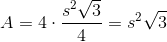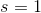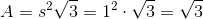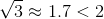# ISEE Upper Level Quantitative : How to find the surface area of a tetrahedron

## Example Questions

### Example Question #32 : Solid Geometry

Which is the greater quantity?

(a) The surface area of a regular tetrahedron with edges of length 1

(b) 2

(a) is greater.

It is impossible to tell from the information given.

(b) is greater.

(a) and (b) are equal.

(b) is greater.

Explanation:

A regular tetrahedron has four faces, each of which is an equilateral triangle. Therefore, its surface area, given sidelength, is.

Substitute:, so (b) is greater.